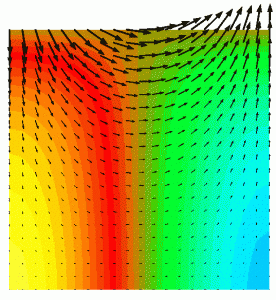Jacek A. Jankowski

PhD thesis Abstract

An algorithm for solution of three-dimensional Navier-Stokes equations for incompressible free surface flows is developed. A decoupled algorithm based on the fractional step (operator-splitting) technique is applied. The solution is obtained in subsequent stages treating equations split into parts having well-defined mathematical properties, so that the most adequate methods for a given differential operator type can be used. The decoupled algorithm structure, which does not use the continuity equation explicitly, allows application of equal-order linear interpolation functions for all variables. The applied reference element type, a prism with six nodes and linear interpolation functions, is a compromise between the exactness of the interpolation, model complexity and computational cost. The finite difference method is applied for the time discretisation and the computational domain variability is taken into account by a standard sigma-mesh structure which is well suited to most geophysical applications.

The model is implemented in the framework of the Telemac system, developed in Laboratoire National d’Hydraulique in Chatou, Electricité de France (EDF) for modelling of free surface flows including transport phenomena. It is a far-reaching further development of existing three-dimensional code Telemac-3D whose application domain is limited due to the hydrostatic approximation and because the free surface position is computed from shallow water equations. Therefore, the vertical acceleration is neglected and the free surface and bottom gradients must be small.A standing wave in a closed basin. Hydrodynamic pressure and velocity vectors

In the new model, the vertical acceleration is taken into account by decomposing the global pressure into its hydrostatic (i.e. barotropic as well as baroclinic) and hydrodynamic components. In consequence, the hydrodynamic pressure is found from a pressure Poisson equation from fractional formulation, whereby the hydrostatic part is computed explicitly from the free surface elevation and density field. The final velocity is obtained under assumption of its incompressibility from the projection of the intermediate result, which is found without hydrodynamic pressure gradients.

The free surface position can be found using height function methods based on the kinematic boundary condition or the free surface conservative equation (vertically integrated continuity equation). In the numerical solution algorithm the method of characteristics, streamline upwind Petrov-Galerkin FEM, or explicit formulation in finite elements can be chosen.

The developed new algorithm steps have been verified separately, using analytical solutions. The verification of the algorithm as a whole has been performed using benchmark test cases covering the targeted model application domain. Some of these allowed a formal comparison with an analytical problem solution. They include free surface and internal waves, sub- and supercritical channel flow over a steep ramp, wind- and buoyancy-driven currents.

The implementation of the model within the well-validated Telemac system allows immediate application of the newly developed algorithm for practical hydraulic engineering problems and geophysical flows.

Reference: Jacek A. Jankowski, (1999) A non-hydrostatic model for free surface flows. PhD Thesis (Dissertation), Bericht Nr. 56/1999, Institut für Strömungsmechanik und ERiB, Universität Hannover.